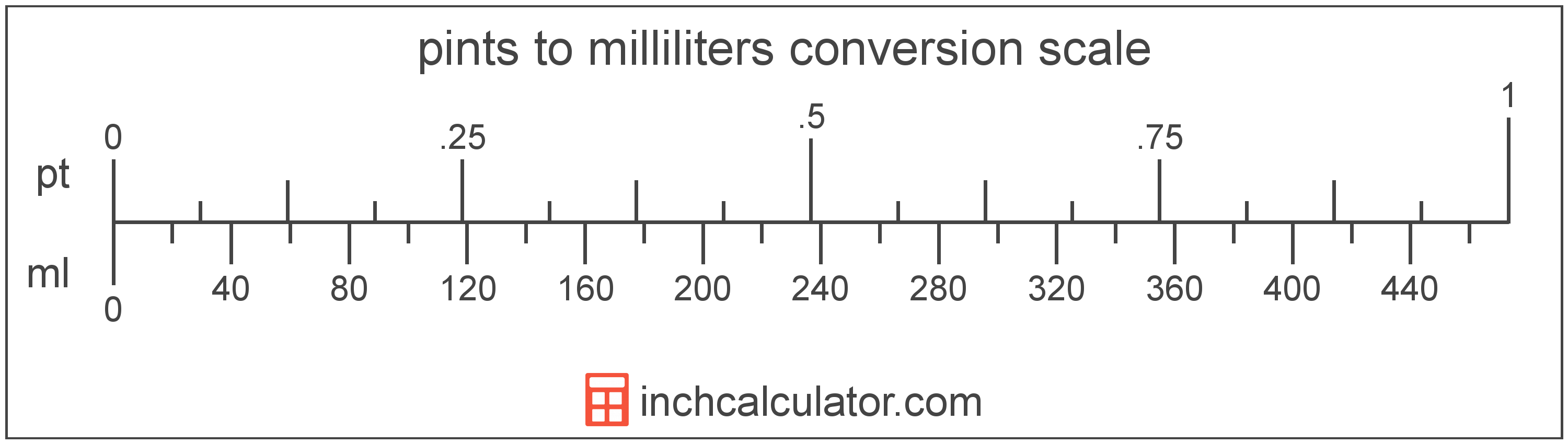# Milliliters to Pints Conversion

Enter the volume in milliliters below to get the value converted to pints.

Results in Pints:1 ml = 0.002113 pt

## How to Convert Milliliters to PintsTo convert a milliliter measurement to a pint measurement, multiply the volume by the conversion ratio.

Since one milliliter is equal to 0.002113 pints, you can use this simple formula to convert:

pints = milliliters × 0.002113

The volume in pints is equal to the milliliters multiplied by 0.002113.

For example, here's how to convert 500 milliliters to pints using the formula above.
500 ml = (500 × 0.002113) = 1.056688 pt

## Milliliters

The milliliter is a unit of volume equal to 1 cubic centimeter, 1/1,000 of a liter, or about 0.061 cubic inches.

The milliliter is an SI unit of volume in the metric system. In the metric system, "milli" is the prefix for 10-3. A milliliter is sometimes also referred to as a millilitre. Milliliters can be abbreviated as ml, and are also sometimes abbreviated as mL or mℓ. For example, 1 milliliter can be written as 1 ml, 1 mL, or 1 mℓ.

Milliliters are sometimes expressed using the abbreviation cc for medical dosage, which is the abbreviation for the cubic centimeter.

## Pints

The US liquid pint is a unit of fluid volume equal to one-eighth of a gallon, one-half of a quart, or two cups. The liquid pint should not be confused with the dry pint (US) or the imperial pint, which are different units.

The pint is a US customary unit of volume. Pints can be abbreviated as pt; for example, 1 pint can be written as 1 pt.

## Milliliter to Pint Conversion Table

Milliliter measurements converted to pints
Milliliters Pints
1 ml 0.002113 pt
2 ml 0.004227 pt
3 ml 0.00634 pt
4 ml 0.008454 pt
5 ml 0.010567 pt
6 ml 0.01268 pt
7 ml 0.014794 pt
8 ml 0.016907 pt
9 ml 0.01902 pt
10 ml 0.021134 pt
20 ml 0.042268 pt
30 ml 0.063401 pt
40 ml 0.084535 pt
50 ml 0.105669 pt
60 ml 0.126803 pt
70 ml 0.147936 pt
80 ml 0.16907 pt
90 ml 0.190204 pt
100 ml 0.211338 pt
200 ml 0.422675 pt
300 ml 0.634013 pt
400 ml 0.845351 pt
500 ml 1.0567 pt
600 ml 1.268 pt
700 ml 1.4794 pt
800 ml 1.6907 pt
900 ml 1.902 pt
1,000 ml 2.1134 pt

## References

1. National Institute of Standards and Technology, Specifications, Tolerances, and Other Technical Requirements for Weighing and Measuring Devices, Handbook 44 - 2019 Edition, https://nvlpubs.nist.gov/nistpubs/hb/2019/NIST.HB.44-2019.pdf